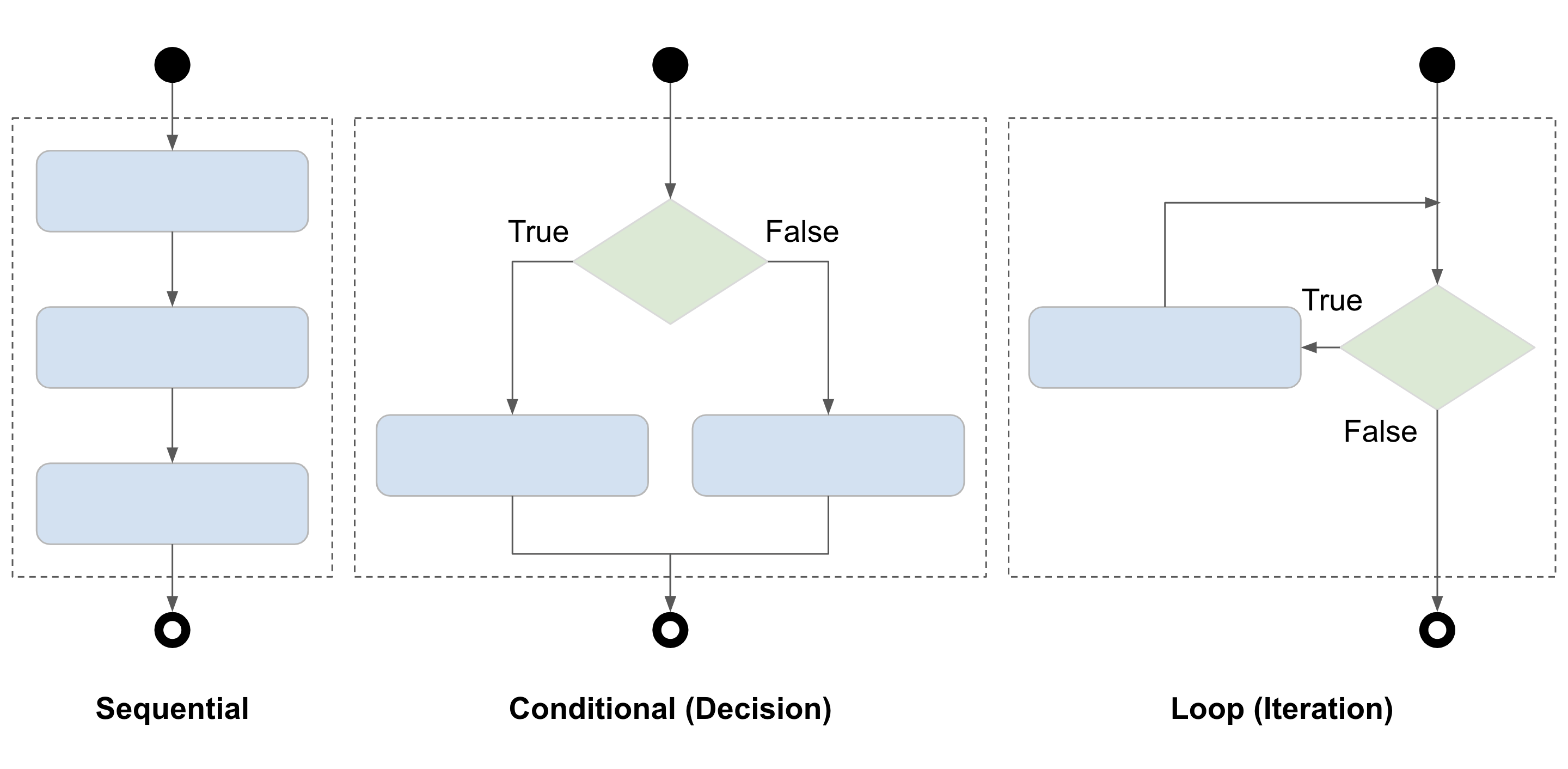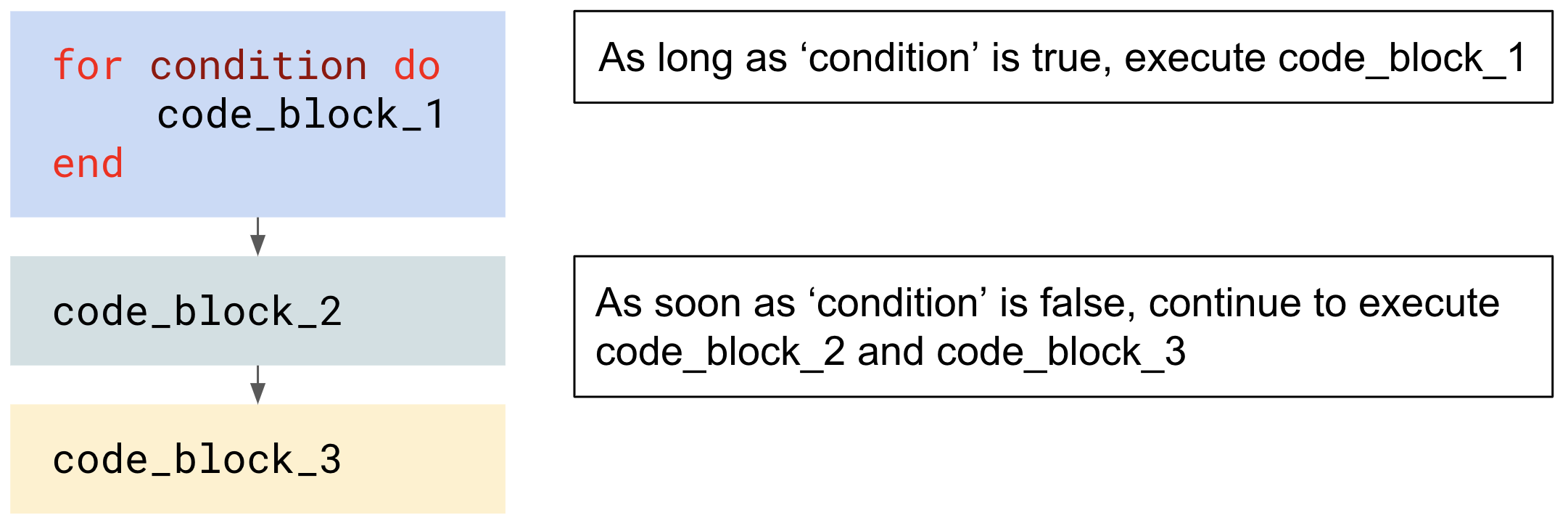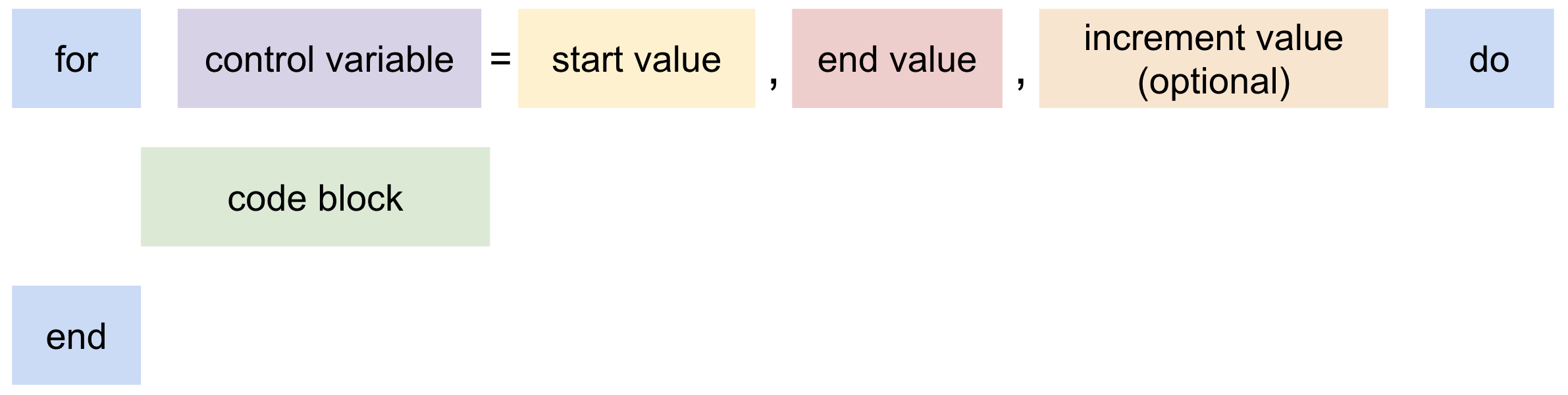# Control structures

A control structure is a block of programming that analyses variables and chooses a direction in which to go based on given parameters. The term “flow control” describes the direction the program takes (which way program control "flows") - this makes it the basic decision-making process in computing.Sequential, conditional, and loops are different types of control structures.

## Conditional statements​

Conditional statements cause programs to perform actions when specific conditions are met. These conditions can be defined by using the following conditional operators:

OperatorDescription
==Equal to
~=Not equal to
>Greater than
<Less than
>=Greater than or equal to
<=Less than or equal to

### `if...then`​

This executes a block of code if a certain condition is true.

``function onSensorEnter(object)    if object.isPlayer == true then        Door.open()    end               <-- Closes the if...then conditional statementend                 <-- Closes the onSensorEnter() function``

When the above program runs:

1. Whenever an object enters the sensor of a door, SCS calls the global event function `onSensorEnter()` , and passes `object` as an input (which represents the object that triggered the sensor).
2. The `if...then` conditional statement checks if the object is a Player.
3. If the object is a Player, the door is opened by calling the `open()` function from the `Door` module.

### `elseif...then`​

This can be inserted into an `if ... then` statement to check for alternative conditions. The program will run from top to bottom and execute the code connected to the first true condition that it encounters.

``function onSensorEnter(object)    if object.isPlayer == true then        Door.open()    elseif object.isEntity == true then        Door.open()    end               <-- Closes the if...then conditional statementend                 <-- Closes the onSensorEnter() function``

When the above program runs:

1. Whenever an object enters the sensor of a door, SCS calls the global event function `onSensorEnter()` , and passes `object` as an input (which represents the object that triggered the sensor).
2. The `if...then` conditional statement checks if the object is a Player.
3. If the object is a Player, the door is opened by calling the `open()` function from the `Door` module.
4. If the object is not a Player, the `elseif...then` conditional statement checks if the object is an Entity.
5. If the object is an Entity, the door is closed by calling the `open()` function from the `Door` module.

### `else`​

This can be inserted to execute a block of code when all preceding conditions are false.

``function onSensorEnter(object)    if object.isPlayer == true then        Door.open()    elseif object.isEntity == true then        Door.open()    else        Door.close()    end               <-- Closes the if...then conditional statementend                 <-- Closes the onSensorEnter() function``

When the above program runs:

1. Whenever an object enters the sensor of a door, SCS calls the global event function `onSensorEnter()` , and passes `object` as an input (which represents the object that triggered the sensor).
2. The `if...then` conditional statement checks if the object is a Player.
3. If the object is a Player, the door is opened by calling the `open()` function from the `Door` module.
4. If the object is not a Player, the `elseif...then` conditional statement checks if the object is an Entity.
5. If the object is an Entity, the door is closed by calling the `open()` function from the `Door` module.
6. If the object is neither a Player nor an Entity, the code in the `else` conditional statement will run, closing the door by calling the `close()` function from the `Door` module.

## Loops​

A loop allows you to run a block of code multiple times. A loop can be written to run a block of code a specific number of times, while a specific condition is true, or indefinitely.

When a loop stops running, the program will continue to execute the code immediately following the loop.In Lua, there are three types of loop; `for`, `while`, and `repeat`.

### `for...do`​

The basic syntax for the `for` loop includes a control variable, a start value, an end value, and an optional increment value.The `for` loop checks if the value of a “control variable” is less-than or equal-to an “end value”. If true, the value of the control variable will be incremented by the specified “increment value” (usually 1), and the code between `do` and `end` will execute. If false, the loop will end.

``for count = 1, 5, 1 do    print(count)end``

When the above loop runs; 1, 2, 3, 4, and 5 are printed on individual lines to the log console.

### `while...do`​

The `while` loop checks if a condition is true or false. If it is true, the code between `do` and `end` will execute and the true/false condition will then be checked again afterward. If it is false, the loop will end.

``local count = 1while count <= 5 do    print(count)    count = count + 1end``

When the above loop runs; 1, 2, 3, 4, and 5 are printed on individual lines to the log console.

### `repeat...until`​

`repeat` loop repeats until a certain condition is met. Unlike other loops, the conditional test in a `repeat` loop is performed after each iteration of the loop. This means the code between `repeat` and `until` is executed at least once, because the conditional test is performed after the code is executed.

``local count = 1repeat    print(count)    count = count + 1until (count > 5)``

When the above loop runs; 1, 2, 3, 4, and 5 are printed on individual lines to the log console.